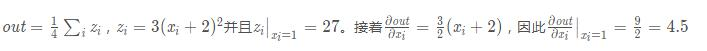variables（变量的序列） - 被求微分的叶子节点，即 ys 。

create_graph（bool，可选） - 如果为True，则将构造派生图，允许计算更高阶的派生产品。默认为False。

```import torch
y = x + 2
z = y * y * 3
out = z.mean()
out.backward()#这里是默认情况，相当于out.backward(torch.Tensor([1.0]))

```Variable containing:
4.5000 4.5000
4.5000 4.5000
[torch.FloatTensor of size 2x2]``````x = torch.randn(3)

y = x * 2
while y.data.norm() < 1000:
y = y * 2

```

```Variable containing:
204.8000
2048.0000
0.2048
[torch.FloatTensor of size 3]```